# RandomYang

Code + Design => Magic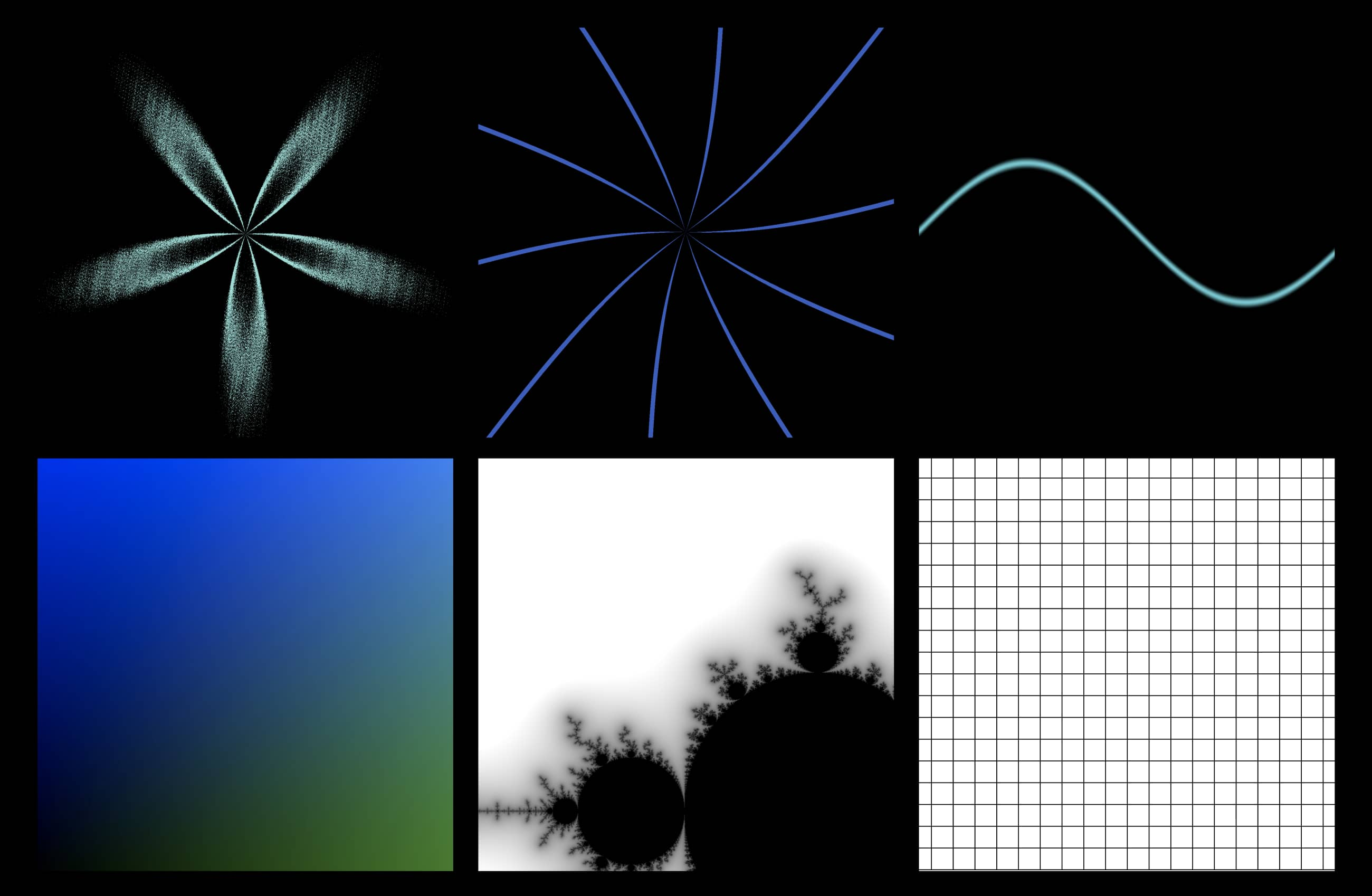## 基础知识(熟悉可跳过)

• 编写着色器程序用到的一门编程语言叫做glsl，语法和C系语言很像，且是强类型。
• 一些常用的基本类型：int整型，float浮点数，vec(2/3/4)分别表示二维、三维、四维向量。(本文涉及到最多的是vec2)。
• uniform变量：
uniform [变量类型] [变量名称]

• 全局变量

• gl_FragCoord：该变量由运行环境给出，表示当前坐标
• gl_FragColor：该变量由运行环境给出，通常会被赋值为最终输出的颜色

## 分类实例

### Color Map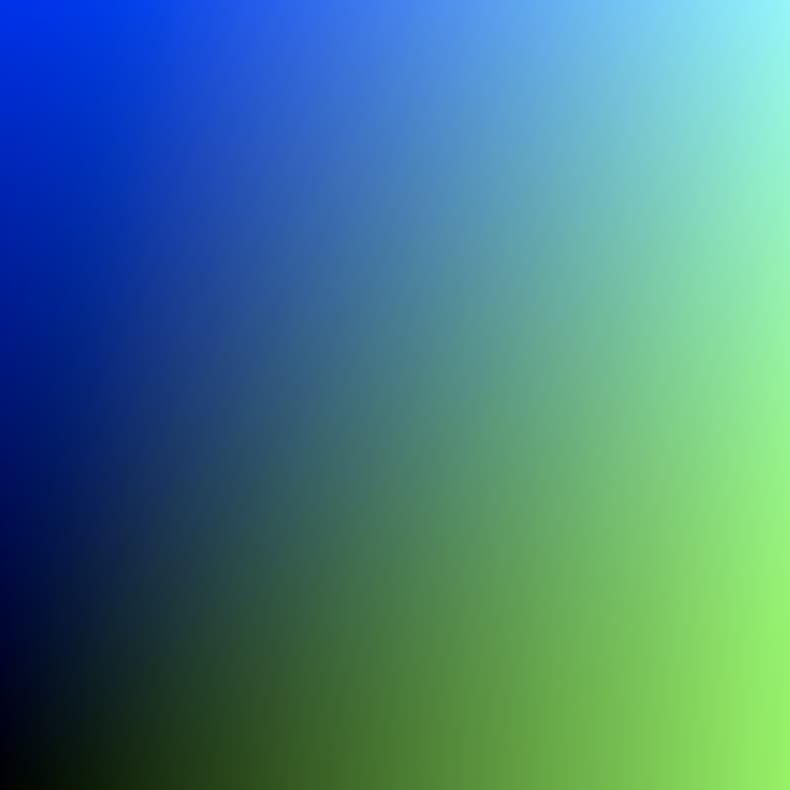precision highp float; // 声明float的精度

uniform vec2 u_size;   // 当前画布的尺寸

void main() {
vec2 rg_size = gl_FragCoord.xy / u_size; // x,y分量限制在[0,1]
gl_FragColor = vec4(
0.0,
rg_size.x,
rg_size.y,
1.0
);
}

gl_FragCoord表示的是当前片元着色器处理的片元(暂时理解为像素)的坐标，是一个vec4类型的变量(x, y, z, 1/w)，目前你只需要知道gl_FragCoord.xy表示取出第1和2两个分量，分别表示水平坐标x和纵坐标y(坐标原点位于左下角)。

### 三角函数曲线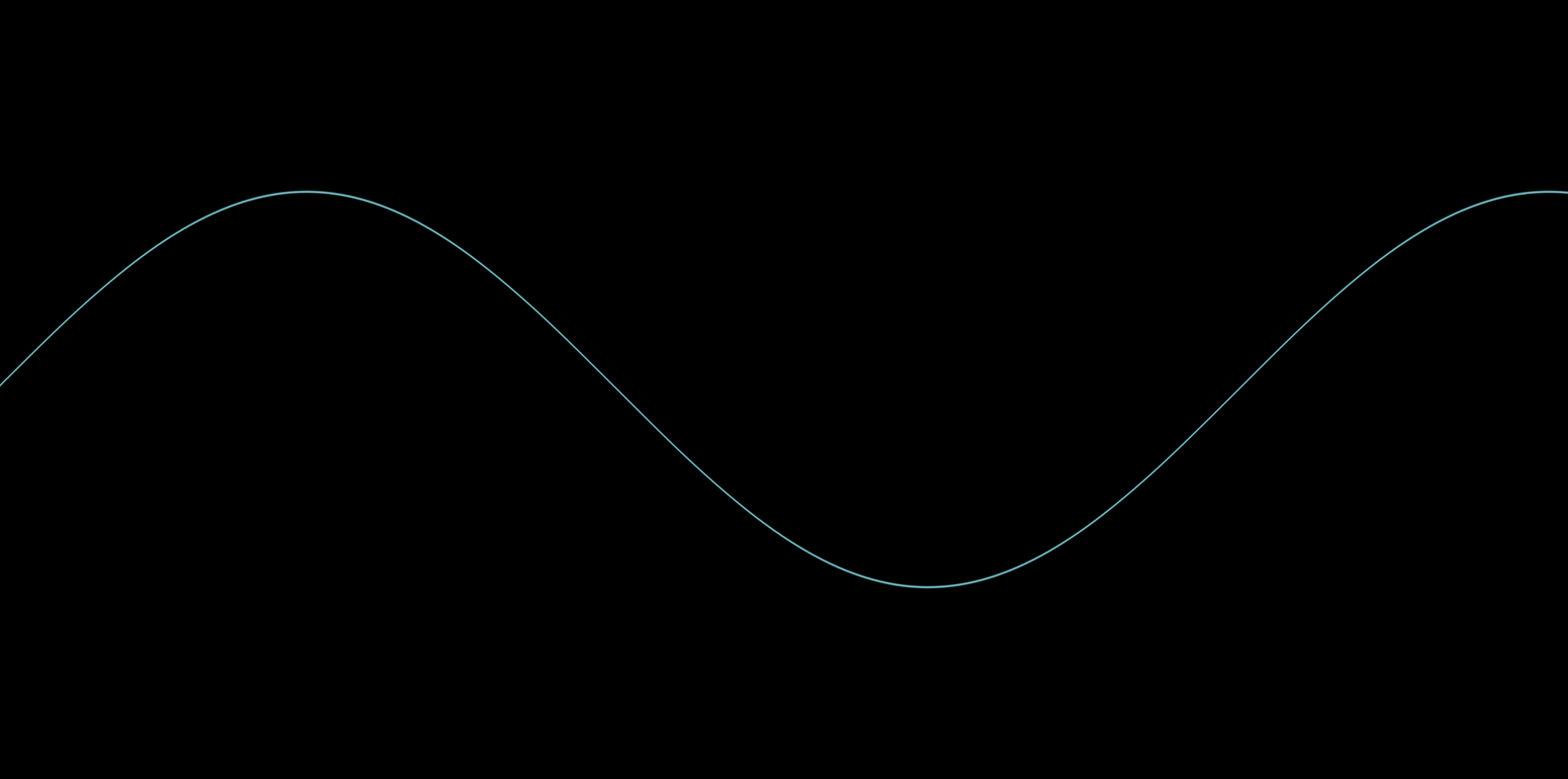precision highp float; // 声明float的精度
#define PI 3.14159265359

uniform vec2 u_size;   // 当前画布的尺寸
uniform float u_dpr;   // 绘制环境的devicePixelRatio

// 定义一个函数
// value 待测值
// target 目标值
float smoothstep_filter(float value, float target){
return smoothstep(target - 0.01, target, value) - smoothstep(target, target + 0.01, value);
}

void main() {
float rate = 100.0 * u_dpr;
vec2 st = gl_FragCoord.xy / vec2(rate);               // 减小画布坐标数值范围
vec3 color = vec3(0.0);                               // 黑色背景
vec3 line_color = vec3(38.0, 204.0, 213.0) / vec3(255.0);

float y = sin(st.x) + (u_size.y / rate) * 0.5;        // 一个周期为2PI, 振幅为1的sin三角函数
// float y = tan(st.x) + (u_size.y / rate) * 0.5;     // 一个周期为PI的tan三角函数

float percent = smoothstep_filter(st.y, y);
color = mix(color, line_color, percent);

gl_FragColor = vec4(color, 1.0);
}

### 极坐标曲线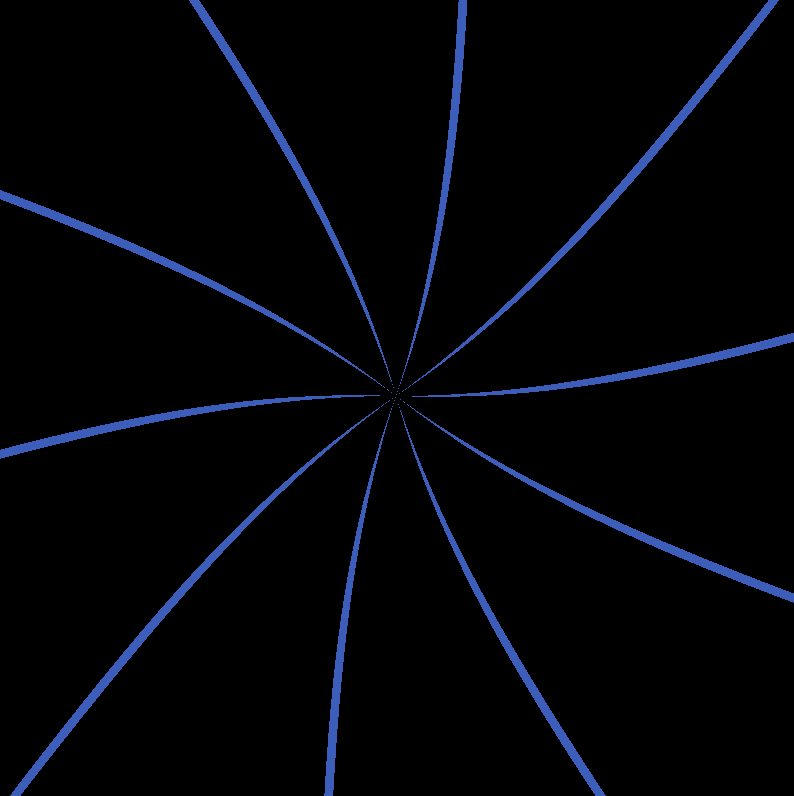precision highp float; // 声明float的精度
#define PI 3.14159265359

uniform vec2 u_size;   // 当前画布的尺寸
uniform float u_dpr;   // 绘制环境的devicePixelRatio

// 根据输入的value和目标value
// 返回位于目标点[0.0, +half_pi]范围内的突变值
float step_filter(float value, float taget_value){
return step(taget_value, value) -
step(taget_value + 0.2, value);
}

// color pattern
vec3 color_1 = vec3(45.0, 89, 198) / vec3(255.0);
vec3 color_2 = vec3(49, 142, 222) / vec3(255.0);
vec3 color_3 = vec3(38, 205, 213) / vec3(255.0);
vec3 color_4 = vec3(118, 224, 214) / vec3(255.0);

void main() {
vec2 st = gl_FragCoord.xy/u_size.xy;
vec3 color = vec3(0.0);

vec2 pos = vec2(0.5) - st;                        // 中心指向当前坐标点的向量
float distance = length(pos)*2.0;                 // 中心点到当前坐标点的距离

float alpha = atan(pos.y,pos.x);                  // 当前位置对应的旋转角
float f = tan(alpha * 5.);                        // 当前角度对应的函数值

color = mix(color, color_1, step_filter(distance, f));  // 比较实际距离 distance 和函数值 f 的大小
gl_FragColor = vec4(color, 1.0);                        // 进而影响当前像素的最终颜色
}

### 噪声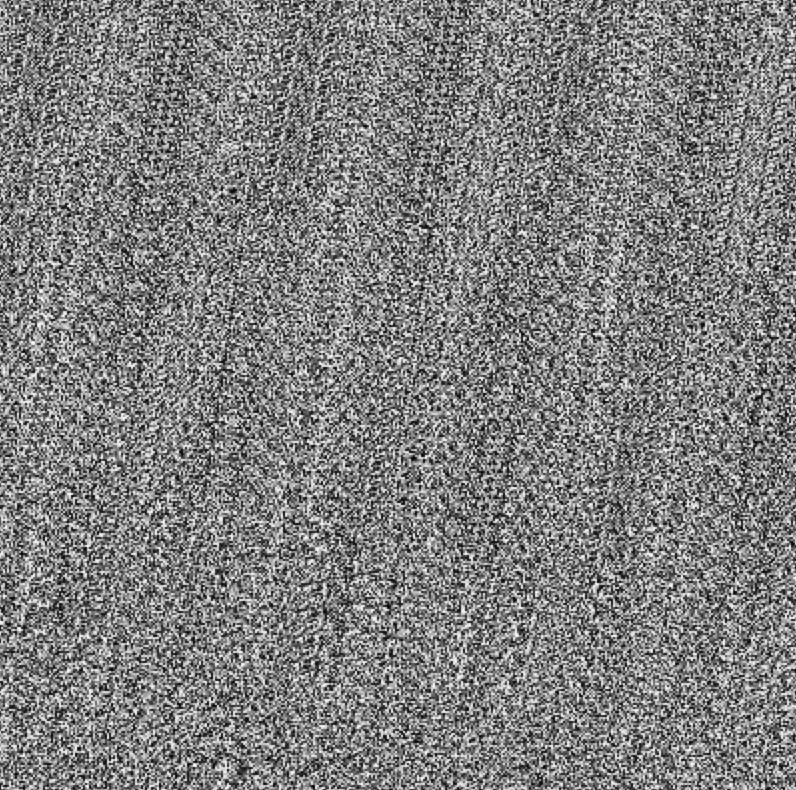precision highp float; // 声明float的精度
#define PI 3.14159265359

uniform vec2 u_size;   // 当前画布的尺寸

// 2D Random
float random (in vec2 st) {
return fract(sin(dot(st.xy,
vec2(12.9898,78.233)))
* 43758.5453123);
}

// 2D Noise based on Morgan McGuire @morgan3d
float noise (in vec2 st) {
vec2 i = floor(st);
vec2 f = fract(st);

// Four corners in 2D of a tile
float a = random(i);
float b = random(i + vec2(1.0, 0.0));
float c = random(i + vec2(0.0, 1.0));
float d = random(i + vec2(1.0, 1.0));

// Smooth Interpolation

// Cubic Hermine Curve.  Same as SmoothStep()
vec2 u = f*f*(3.0-2.0*f);
// u = smoothstep(0.,1.,f);

// Mix 4 coorners percentages
return mix(a, b, u.x) +
(c - a)* u.y * (1.0 - u.x) +
(d - b) * u.x * u.y;
}

void main() {
vec2 st = gl_FragCoord.xy/u_size.xy;

// Scale the coordinate system to see
// some noise in action
vec2 pos = vec2(st*400.0);

// Use the noise function
float n = noise(pos);

gl_FragColor = vec4(vec3(n), 1.0);
}

### 分形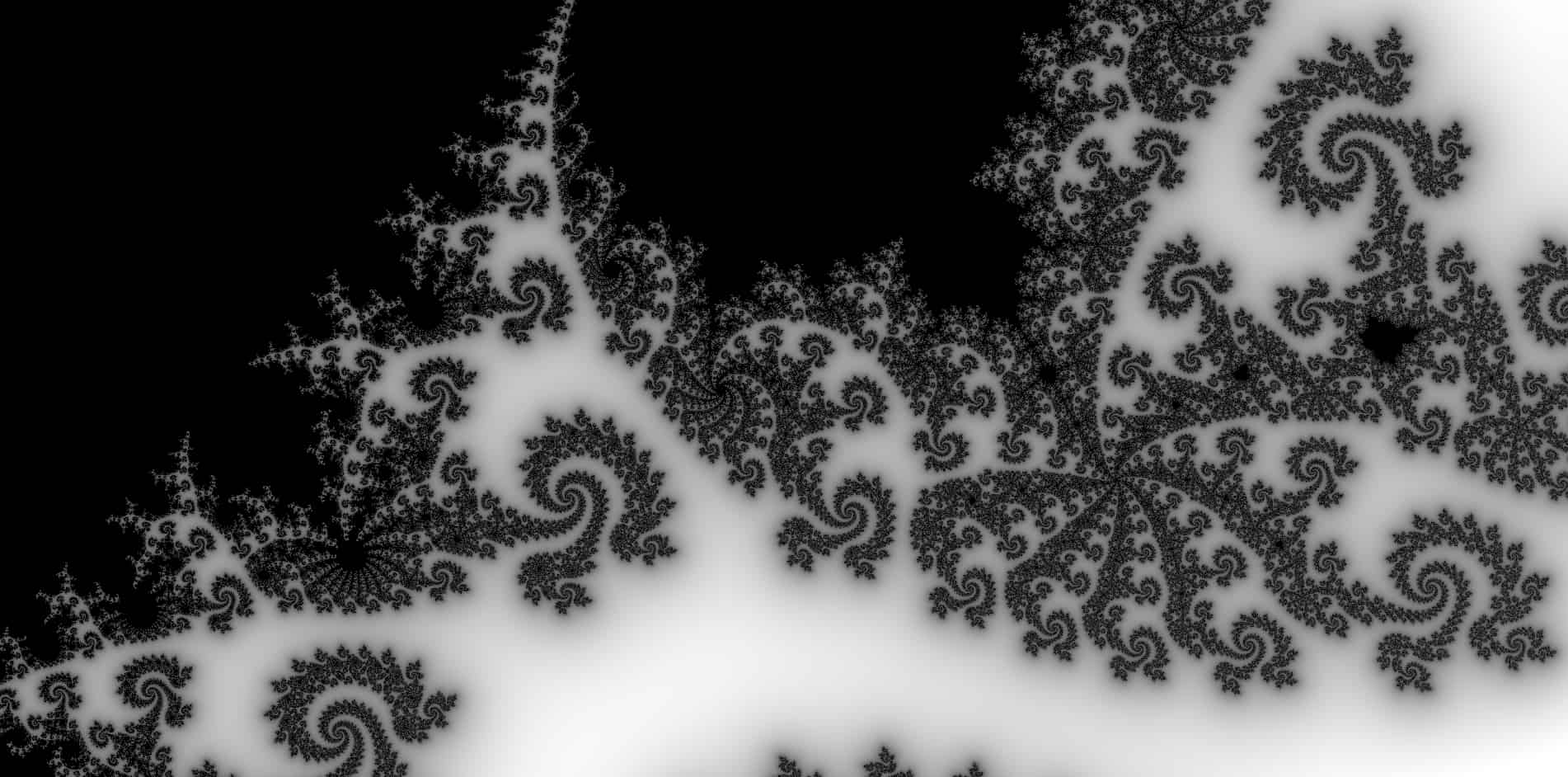// https://www.shadertoy.com/view/lsX3W4

precision mediump float;

uniform vec2 u_size;
uniform float u_time;
float fixedTime = u_time / 2.0;

float distanceToMandelbrot(in vec2 c)
{
#if 1
{
float c2 = dot(c, c);
// skip computation inside M1 - http://iquilezles.org/www/articles/mset_1bulb/mset1bulb.htm
if (256.0 * c2 * c2 - 96.0 * c2 + 32.0 * c.x - 3.0 < 0.0)
return 0.0;
// skip computation inside M2 - http://iquilezles.org/www/articles/mset_2bulb/mset2bulb.htm
if (16.0 * (c2 + 2.0 * c.x + 1.0) - 1.0 < 0.0)
return 0.0;
}
#endif

// iterate
float di = 1.0;
vec2 z = vec2(0.0);
float m2 = 0.0;
vec2 dz = vec2(0.0);
for (int i = 0; i < 300; i++)
{
if (m2 > 1024.0)
{
di = 0.0;
break;
}

// Z' -> 2·Z·Z' + 1
dz = 2.0 * vec2(z.x * dz.x - z.y * dz.y, z.x * dz.y + z.y * dz.x) + vec2(1.0, 0.0);

// Z -> Z² + c
z = vec2(z.x * z.x - z.y * z.y, 2.0 * z.x * z.y) + c;

m2 = dot(z, z);
}

// distance
float d = 0.5 * sqrt(dot(z, z) / dot(dz, dz)) * log(dot(z, z));
if (di > 0.5)
d = 0.0;

return d;
}

void main()
{
vec2 p = (2.0 * gl_FragCoord.xy - u_size.xy) / u_size.y;

// animation
float tz = 0.5 - 0.5 * cos(0.225 * fixedTime);
float zoo = pow(0.5, 13.0 * tz);
vec2 c = vec2(-0.05, .6805) + p * zoo;

// distance to Mandelbrot
float d = distanceToMandelbrot(c);

// do some soft coloring based on distance
d = clamp(pow(4.0 * d / zoo, 0.2), 0.0, 1.0);

vec3 col = vec3(d);

gl_FragColor = vec4(col, 1.0);
}

### 图形pattern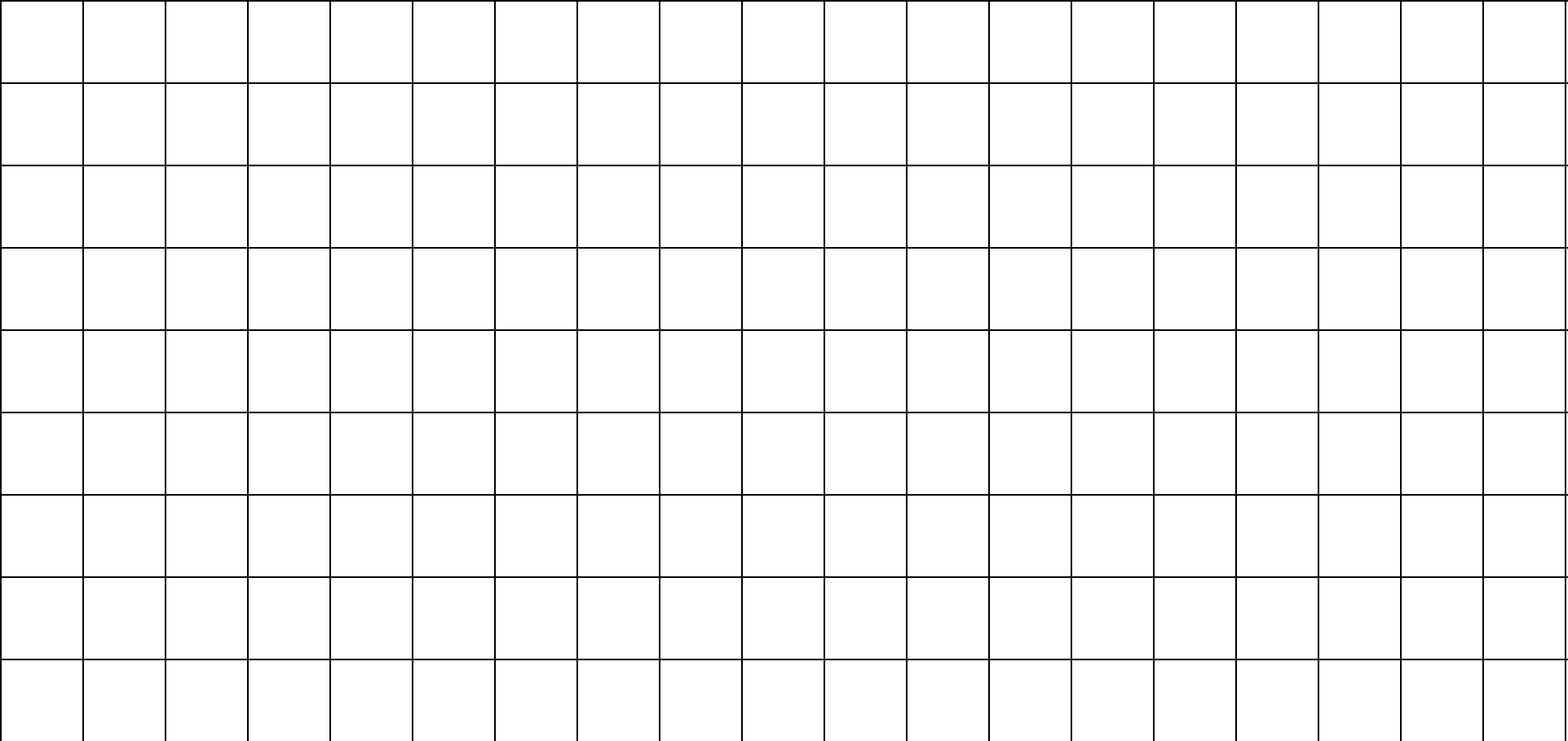precision mediump float;

uniform vec2 u_size;
uniform float u_time;
float fixedTime = u_time / 2.0;

float width_1 = 2.0;
float width_2 = 2.0;
float f_x = 100.0;
float f_y = 100.0;

void main()
{
vec3 color = vec3(1.0);

// x方向上满足
if(mod(gl_FragCoord.x, f_x) <= width_1) {
color = vec3(0.0);
}
// y方向上满足
if(mod(gl_FragCoord.y, f_y) <= width_2) {
color = vec3(0.0);
}

gl_FragColor = vec4(color, 1.0);
}

pattern通常是由周期性重复的图案组成，这里的例子很简单，周期性最小重复单元可以看作是一个黑色的方框。

### 时间系数

precision highp float; // 声明float的精度

// 与JS单向通信的变量
uniform vec2 u_size;   // 当前画布的尺寸
uniform float u_time;  // 一个随时间变化的量

void main() {
vec2 rg_size = gl_FragCoord.xy / u_size; // x,y分量限制在[0,1]
gl_FragColor = vec4(
abs(sin(u_time)),  // 加入时间系数
rg_size.x,
rg_size.y,
1.0
);
}

## 彩蛋——组合

### Pattern + 噪声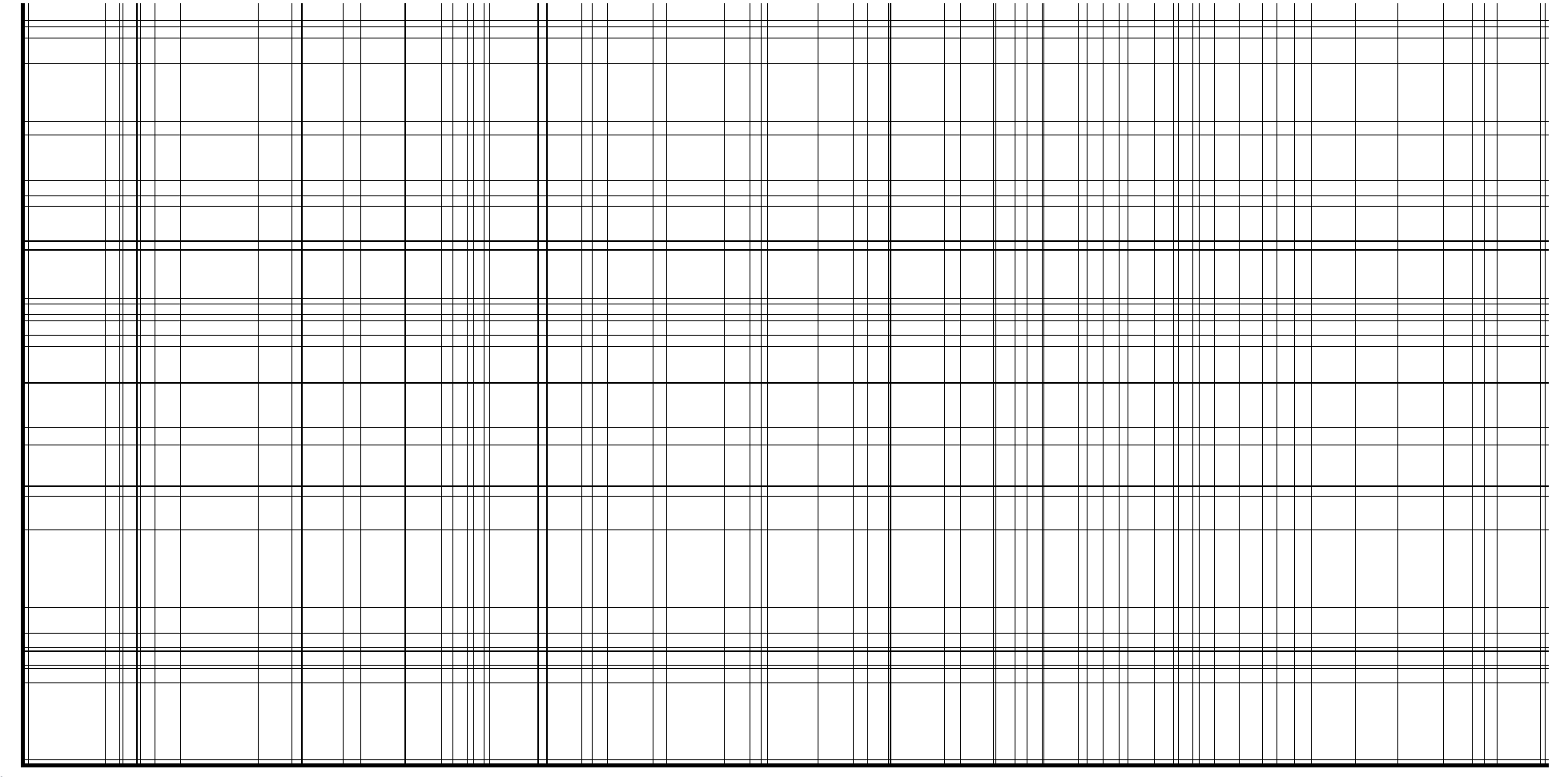### 极坐标曲线 + 噪声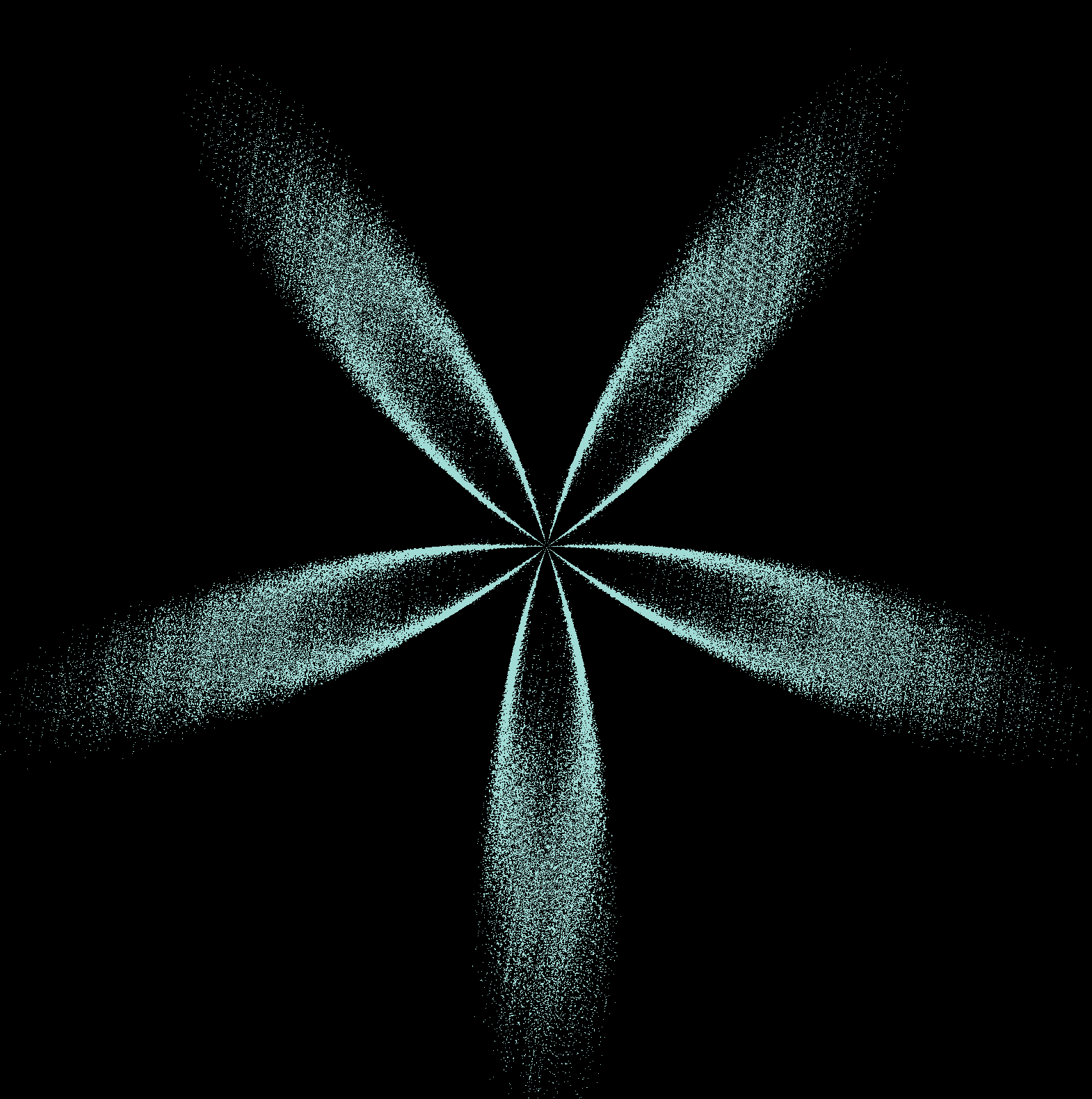### 三角函数 + 噪声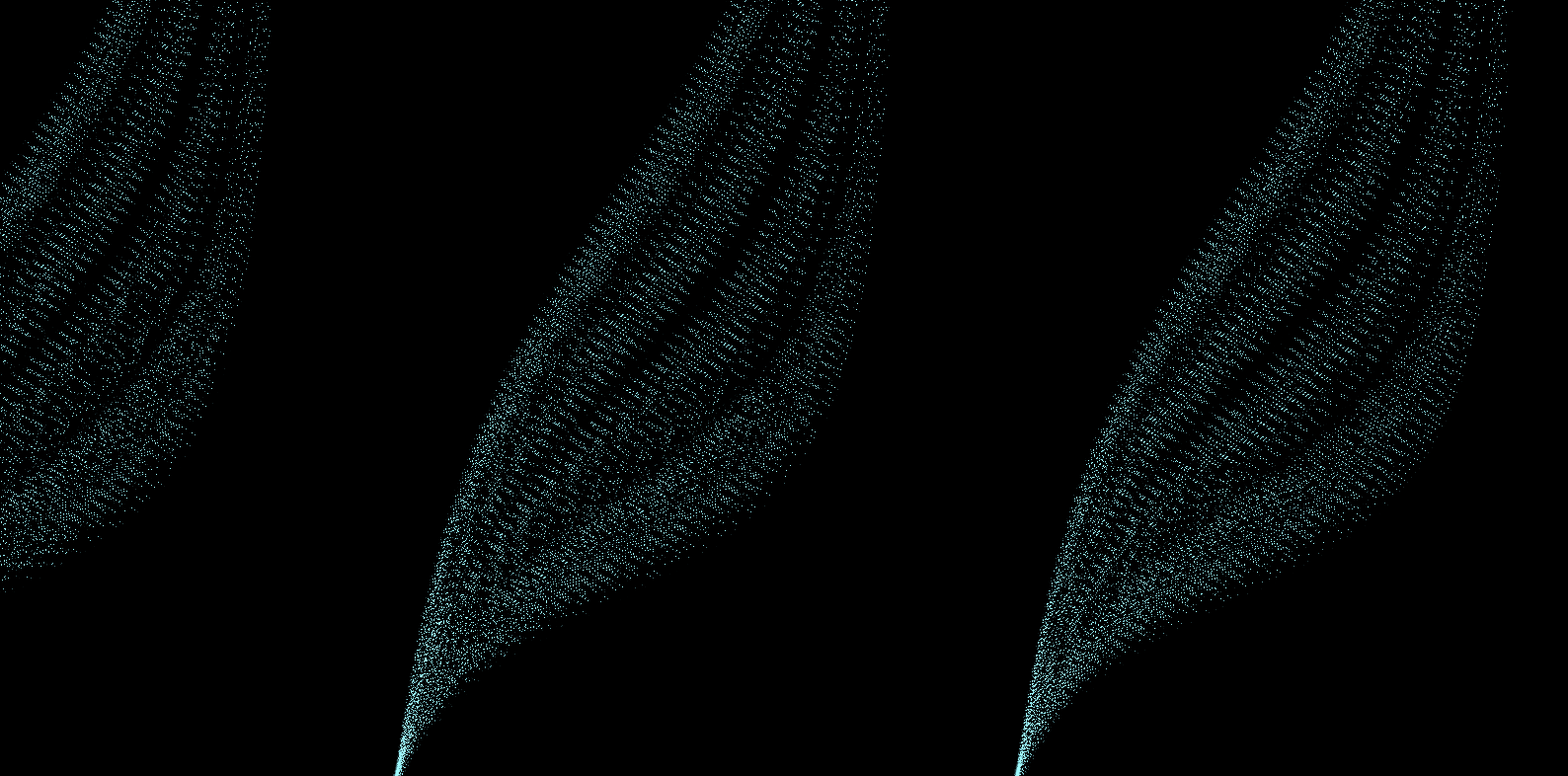### 三角函数 + 过滤函数 +噪声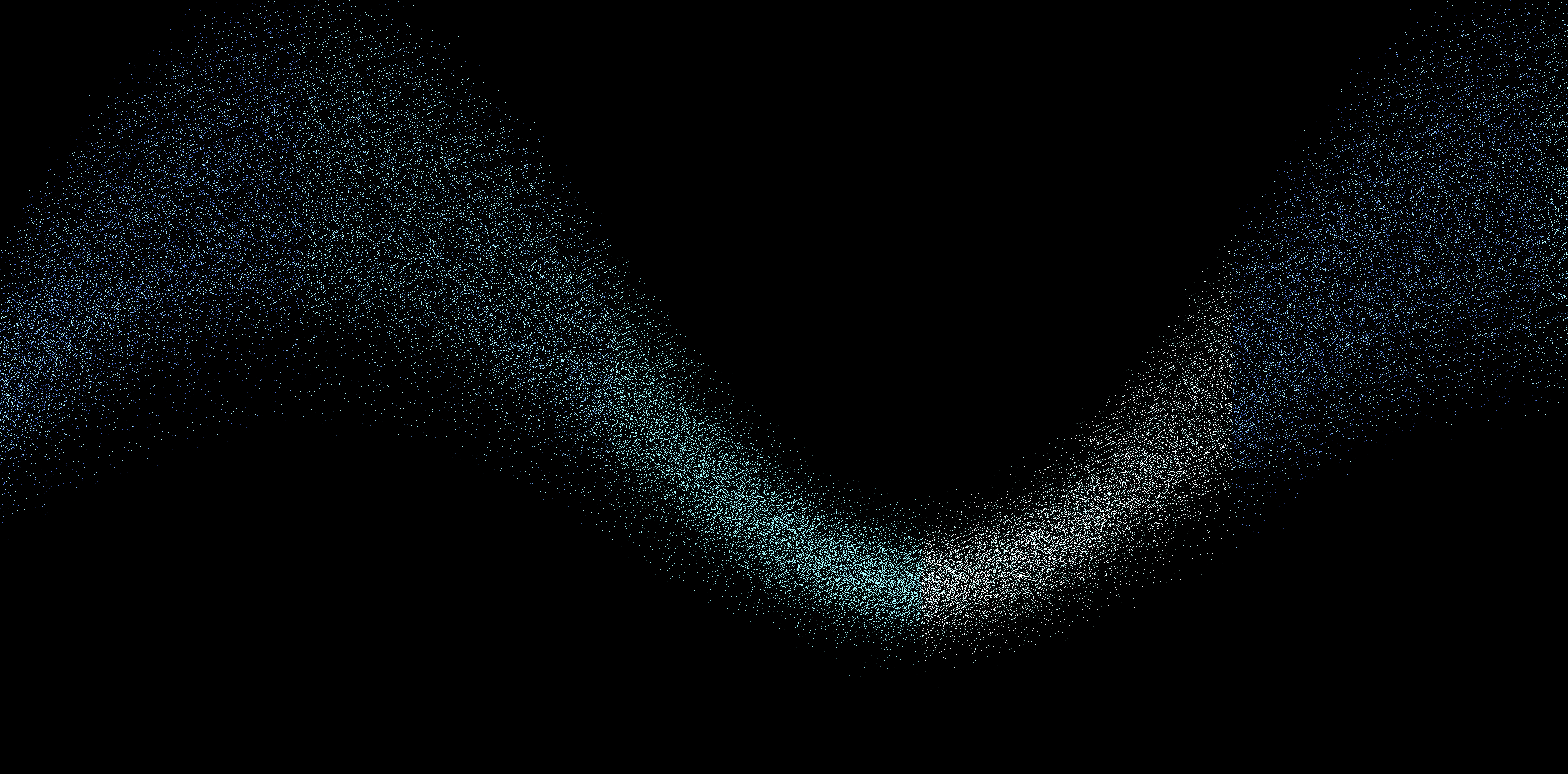ps：这线段上的四种颜色你眼熟吗(狗头)